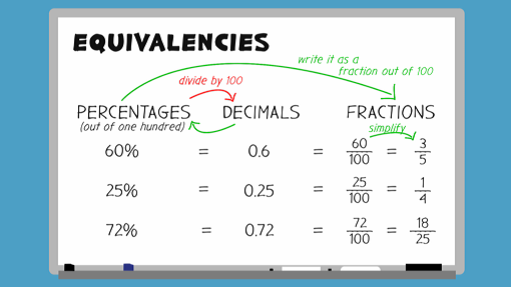Everyday maths 2 (Wales)

Start this free course now. Just create an account and sign in. Enrol and complete the course for a free statement of participation or digital badge if available.

# 9.1 Converting between percentages, decimals and fractions

Since fractions, decimals and percentages are all just different ways of representing the same thing, we can convert between them in order to compare. Take a look at the video below to see how to convert fractions, decimals and percentages.Interactive feature not available in single page view (see it in standard view).

Lets look in more detail at changing a percentage to a fraction.

## Example: 50% is 50 divided by 100

As you can see this percentage is essentially a fraction of 100. However you can simplify it to .

To change a percentage to a fraction, put the percentage over 100 and simplify if possible.

Sometimes we might see a percentage like this: 12.5%.

If we use the method above we get but we can’t have a decimal in a fraction.

To get rid of the decimal in the fraction we must multiply the top and bottom of the fraction, the numerator and denominator, by any number that will give us whole numbers. In this case 10 or 2 both work well (12.5 × 10 = 125 and 12.5 × 2 = 25):

### Method 1: × 10

• × top and bottom by 10 = =

### Method 2: × 2

• × top and bottom by 2 = =

## Activity 21: Converting between percentages, decimals and fractions

1. Express these percentages as decimals:

• a.62%

• b.50%

• c.5%

2. Express these decimals as percentages:

• a.0.02

• b.0.2

• c.0.752

• d.0.055

3. Express these percentages as fractions:

• a.15%

• b.2.5%

• c.37.5%

1.

• a.0.62

• b.0.5

• c.0.05

2.

• a.2%

• b.20%

• c.75.2%

• d.5.5%

3.

• a. =

• b. × top and bottom by 10 = =

• c. × top and bottom by 10 = =

You may have multiplied by different numbers to get rid of the decimal in the last two questions. However, your final answers should still be the same as ours.

Now have a go at matching these fractions to decimals and percentages.

## Activity 22: Matching fractions, decimals and percentages

Choose the correct fraction for each percentage and decimal.

Using the following two lists, match each numbered item with the correct letter.

• a.      62.5% = 0.625 =

• b.      40% = 0.4 =

• c.      8% = 0.08 =

• d.      35% = 0.35 =

• 1 = d
• 2 = b
• 3 = c
• 4 = a

Next you’ll look in more detail at how to change a fraction to a percentage.#### Find out more

*Eligibility rules apply for financial support.